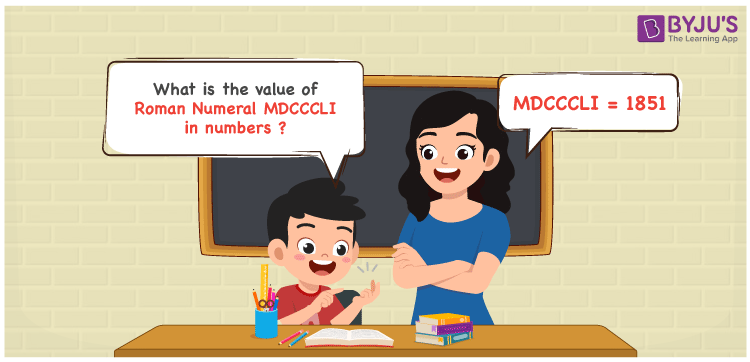Checkout JEE MAINS 2022 Question Paper Analysis : Checkout JEE MAINS 2022 Question Paper Analysis :

# MDCCCLI Roman Numerals

MDCCCLI Roman Numerals is 1851. Roman Numerals are the way of expressing numbers using the Roman alphabet. Students who want to obtain proficiency in converting the Roman Numerals into numbers effortlessly are advised to access Roman Numerals Conversion in PDF format whenever required. In this article, we will learn how to represent the value of MDCCCLI Roman Numerals in numbers with a complete explanation.

 Number Roman Numeral 1851 MDCCCLI

## How to Write MDCCCLI Roman Numerals?The value of MDCCCLI Roman Numerals can be expressed by using any of the two methods given below

First Method:

• Break the Roman Numerals into single letters
• MDCCCLI = M + D + C + C + C + L + I
• Write the numerical value of each letter and add/subtract them
• MDCCCLI = 1000 + 500 + 100 + 100 + 100 + 50 + 1 = 1851

Second Method:

In this method, we consider the groups of Roman Numerals for addition or subtraction

• MDCCCLI = M + DCCC + L + I = 1000 + 800 + 50 + 1 = 1851
• Hence the numerical value of MDCCCLI Roman Numerals is 1851

## Video Lesson on Roman Numerals## Related Articles

Roman Numerals

Roman Numerals 1 to 50

Roman Numerals 1 to 100

Roman Numerals 1 to 1000

L Roman Numerals

Roman Numeral XXXIX

## Frequently Asked Questions on MDCCCLI Roman Numerals

### What is MDCCCLI Roman Numerals?

MDCCCLI Roman Numerals is 1851.

### What is the value of 1800 + 51 in Roman Numerals?

1800 + 51 = 1851. The number 1851 in Roman Numerals is MDCCCLI. Hence the value of 1800 + 51 in Roman Numerals is MDCCCLI.

### How do you read the Roman Numeral MDCCCLI in English?

MDCCCLI Roman Numeral is equal to 1851 and it is read as One Thousand Eight Hundred Fifty-One in English.